# 用动态规划思想简化理解KMP算法8月29日leetcode每日一题是字符串匹配问题，涉及到著名的KMP算法，很早之前就听说过它的鼎鼎大名，查询了点资料现做总结如下，尽量做得简介明了，以供大家参考。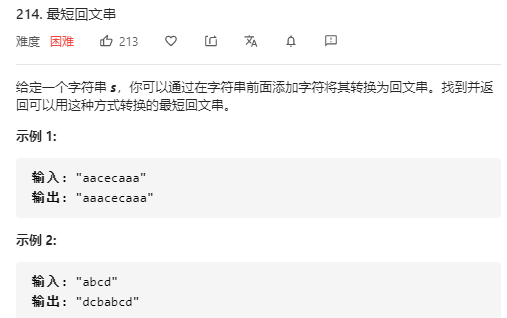KMP 算法（Knuth-Morris-Pratt 算法）是一个著名的字符串匹配算法，效率很高，说实话，有点复杂。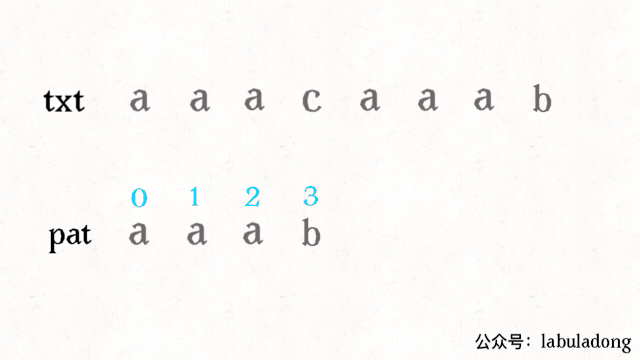KMP 算法的不同之处在于，它会花费空间来记录一些信息，在上述情况中就会显得很聪明。由于pat中根本不存在c字符，所以可以直接跳过这个字符：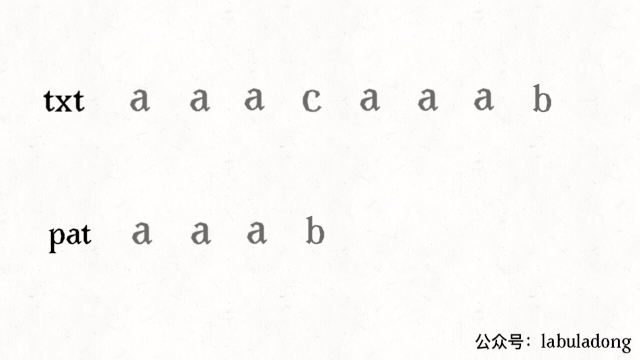KMP 算法则不会重复扫描txt，而是借助next数组中储存的信息把pat移到正确的位置继续匹配，用空间换时间。

``````// KMP算法主体逻辑。str是主串，pattern是模式串
public static int kmp(String str, String pattern) {
//预处理，生成next数组
int[] next = getNexts(pattern);
int j = 0;
//主循环，遍历主串字符
for (int i = 0; i < str.length(); i++) {
while (j > 0 && str.charAt(i) != pattern.charAt(j)) {
//遇到坏字符时，查询next数组并改变模式串的起点
j = next[j];
}
if (str.charAt(i) == pattern.charAt(j)) {
j++;
}
if (j == pattern.length()) {
//匹配成功，返回下标
return i - pattern.length() + 1;
}
}
return -1;
}
// 生成Next数组
private static int[] getNexts(String pattern) {
int[] next = new int[pattern.length()];
int j = 0;
for (int i=2; i<pattern.length(); i++) {
while (j != 0 && pattern.charAt(j) != pattern.charAt(i-1)) {
//从next[i+1]的求解回溯到 next[j]
j = next[j];
}
if (pattern.charAt(j) == pattern.charAt(i-1)) {
j++;
}
next[i] = j;
}
return next;
}
public static void main(String[] args) {
String str = "ATGTGAGCTGGTGTGTGCFAA";
String pattern = "GTGTGCF";
int index = kmp(str, pattern);
System.out.println("首次出现位置：" + index);
}``````

``````public class KMP {
private int[][] dp;
private String pat;

public KMP(String pat) {
this.pat = pat;
// 通过 pat 构建 dp 数组
// 需要 O(M) 时间
}

public int search(String txt) {
// 借助 dp 数组去匹配 txt
// 需要 O(N) 时间
}
}``````

``````KMP kmp = new KMP("aaab");
int pos1 = kmp.search("aaacaaab"); //4
int pos2 = kmp.search("aaaaaaab"); //4``````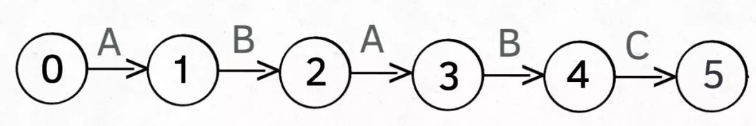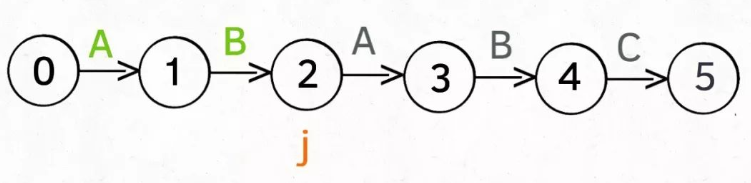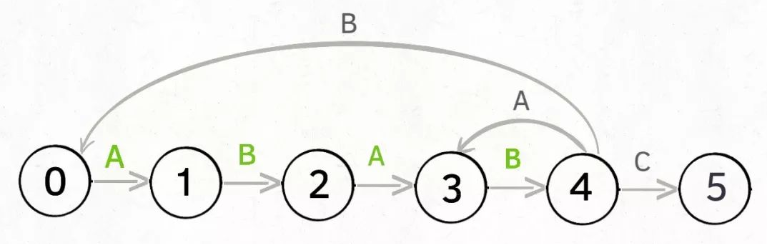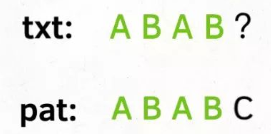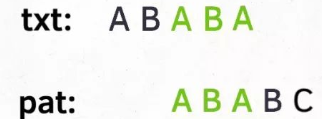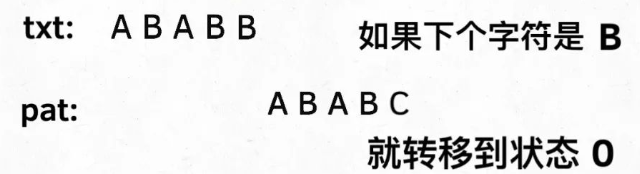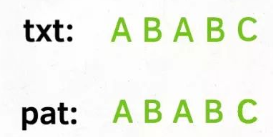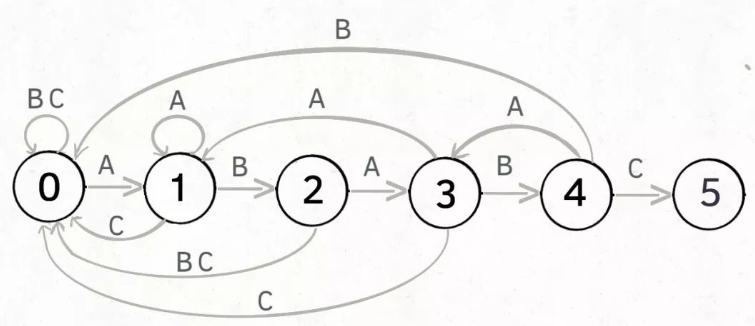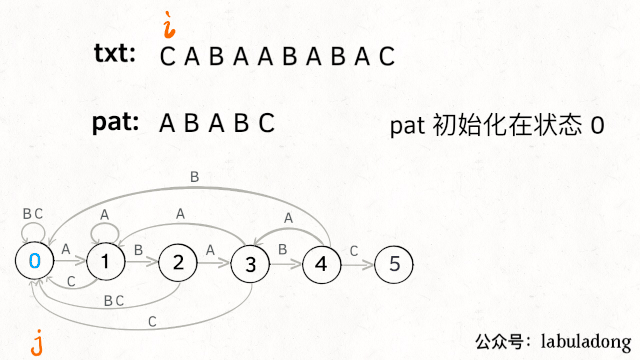``dp[j][c] = next``
• 0 <= j < M，代表当前的状态
• 0 <= c < 256，代表遇到的字符（ASCII 码）
• 0 <= next <= M，代表下一个状态

``dp['A'] = 3``

``dp['B'] = 2``

``````public int search(String txt) {
int M = pat.length();
int N = txt.length();
// pat 的初始态为 0
int j = 0;
for (int i = 0; i < N; i++) {
// 当前是状态 j，遇到字符 txt[i]，
// pat 应该转移到哪个状态？
j = dp[j][txt.charAt(i)];
// 如果达到终止态，返回匹配开头的索引
if (j == M) return i - M + 1;
}
// 没到达终止态，匹配失败
return -1;
}``````

## 3.1 基本框架

“要确定状态转移图，必须明确两个变量，一个是当前的状态，另一个是遇到的字符”。dp数组的构建框架就如下：

``````// 状态
for 0 <= j < M{
// 遇到的字符
for 0 <= c < 256{
dp[j][c] = next;
}
}``````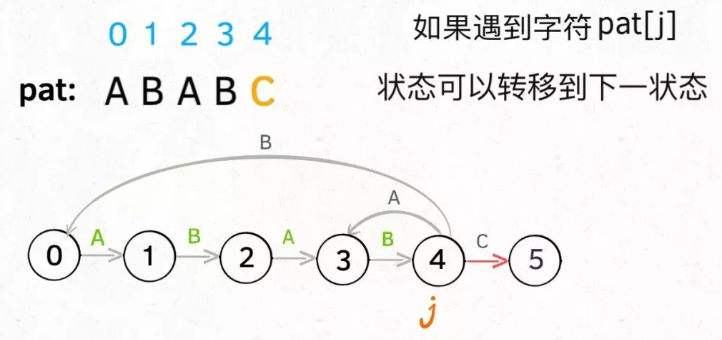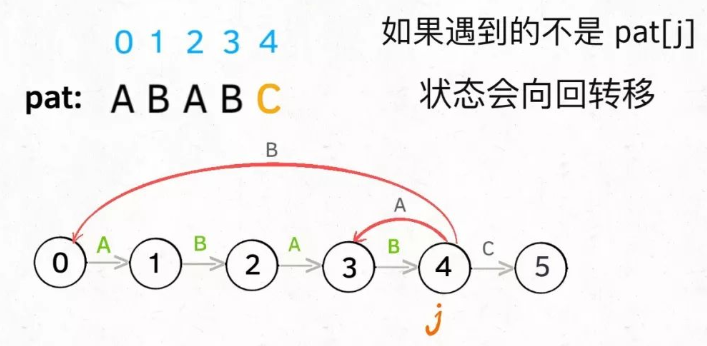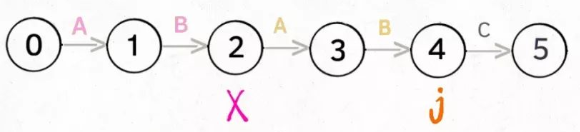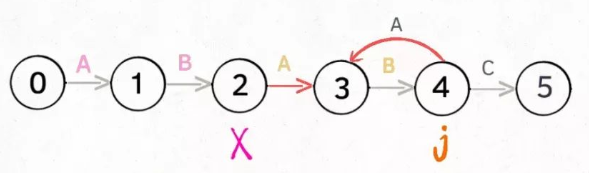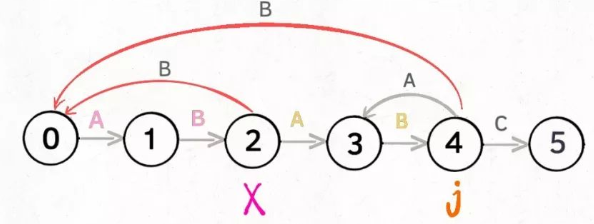X永远跟在j的身后，状态X如何转移，在之前就已经算出来了(动态规划 或者 递归的思想)

``````// 影子状态
int x;
for 0 <= j < M{
// 遇到的字符
for 0 <= c < 256{
if(c == pat[j]){
// 状态推进
dp[j][c] = j + 1;
}else{
// 状态重启
// 利用x计算重启位置
dp[j][c] = dp[x][c];
}
}
}``````

## 3.2 生成影子状态

``````public class KMP {
private int[][] dp;
private String pat;

public KMP(String pat) {
this.pat = pat;
int M = pat.length();
// dp[状态][字符] = 下个状态
dp = new int[M];
// base case
dp[pat.charAt(0)] = 1;
// 影子状态 X 初始为 0
int X = 0;
// 当前状态 j 从 1 开始
for (int j = 1; j < M; j++) {
for (int c = 0; c < 256; c++) {
if (pat.charAt(j) == c)
dp[j][c] = j + 1;
else
dp[j][c] = dp[X][c];
}
// 更新影子状态
X = dp[X][pat.charAt(j)];
}
}

public int search(String txt) {...}
}``````

``dp[pat.charAt(0)] = 1;``

``````int X = 0;
for (int j = 1; j < M; j++) {
...
// 更新影子状态
// 当前是状态 X，遇到字符 pat[j]，
// pat 应该转移到哪个状态？
X = dp[X][pat.charAt(j)];
}``````

``````int j = 0;
for (int i = 0; i < N; i++) {
// 当前是状态 j，遇到字符 txt[i]，
// pat 应该转移到哪个状态？
j = dp[j][txt.charAt(i)];
...
}``````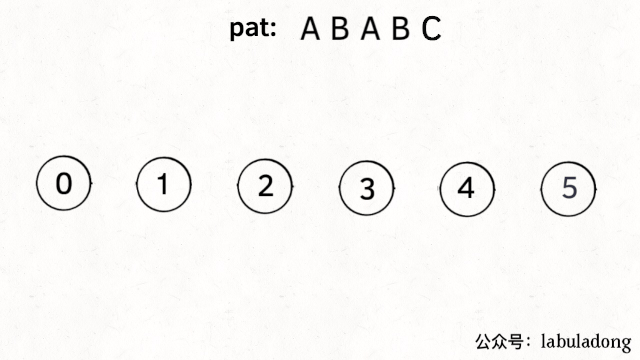``````public class KMP {
private int[][] dp;
private String pat;

public KMP(String pat) {
this.pat = pat;
int M = pat.length();
// dp[状态][字符] = 下个状态
dp = new int[M];
// base case
dp[pat.charAt(0)] = 1;
// 影子状态 X 初始为 0
int X = 0;
// 当前状态 j 从 1 开始
for (int j = 1; j < M; j++) {
for (int c = 0; c < 256; c++) {
if (pat.charAt(j) == c)
dp[j][c] = j + 1;
else
dp[j][c] = dp[X][c];
}
// 更新影子状态
X = dp[X][pat.charAt(j)];
}
}

public int search(String txt) {
int M = pat.length();
int N = txt.length();
// pat 的初始态为 0
int j = 0;
for (int i = 0; i < N; i++) {
// 计算 pat 的下一个状态
j = dp[j][txt.charAt(i)];
// 到达终止态，返回结果
if (j == M) return i - M + 1;
}
// 没到达终止态，匹配失败
return -1;
}
}``````

dp数组的含义：

dpj = next：当前是状态j，遇到了字符c，应该转移到状态next。

-- 展开阅读全文 --

« 上一篇 07-24